• 【目标】使用cocos2d编写一个简单的五子棋程序 【参考】 1、五子棋基本棋型及其特点 2、TestCpp工程 3、五子棋AI循序渐进（虽然是VB，但是的很好） 4、人工智能 （这书其实很一般，我觉得作者对于后面的算法...
【目标】使用cocos2d编写一个简单的五子棋程序

【参考】
1、五子棋基本棋型及其特点
2、TestCpp工程
3、五子棋AI循序渐进（虽然是VB，但是写的很好）
4、人工智能 （这书其实很一般）
5、cococs2d-x 多线程加载plist

9月份颓废了好一阵子，下旬的时候才重新上阵，看到周围的同事在玩五子棋，就忍不住自己也写了一个，写了半个多月。现在算是一个还算拿得出手的小作品。这里总结一下。

一、五子棋游戏框架
作为一个只有一个棋盘和两种棋子的游戏来说，如果单纯只是一个人类选手对战的五子棋平台，那么整个架构比起扫雷来说复杂不了多少。
但是这次却是我写过最复杂的一个程序了，其复杂性主要在于引入了AI。整体的架构如下：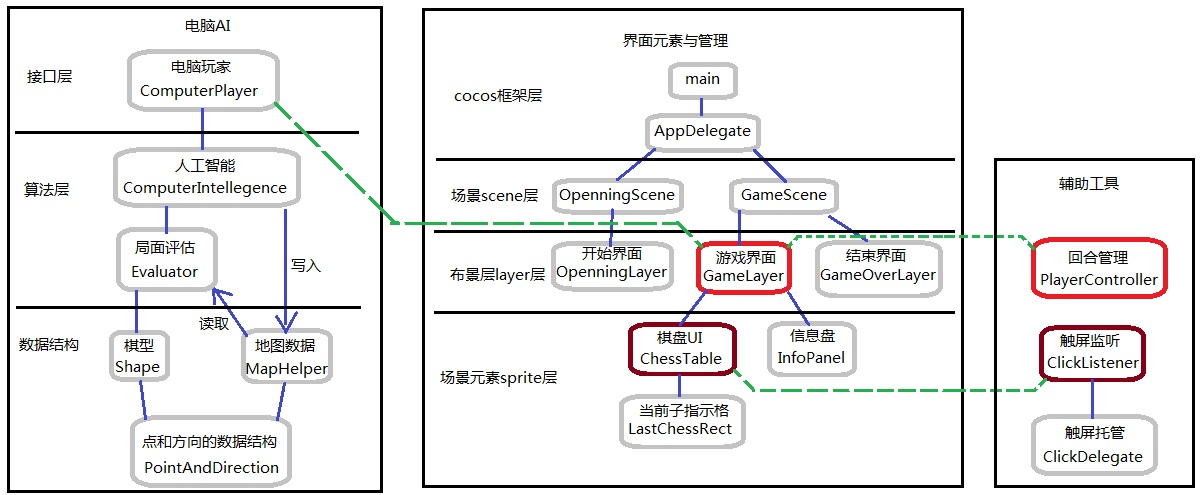对这张图做一些解释：
1、界面部分
抽象分层使用cocos的scene——layer——sprite机制，出于对于逻辑管理与图像处理混搭的恐惧症状，在本部分只集中管理UI，将逻辑处理部分抽象到了辅助层中。
2、辅助工具部分
主要包括两个工具：
一是回合管理。五子棋是两个棋手轮流下，途中可能发生走棋、悔棋、投降、胜利等等情况。另外开始的时候还要设置两个棋手的身份：是不是AI？总之，将这一部分的逻辑处理集中到了 playerController中，而 Gamelayer 继承了这个类，是在实际游戏过程中的回合管理者。
二是触屏管理。在“8.自己的触屏管理——添加ClickManager”里面我就提到过这个东西。这次总体变化不大，只是优化了一下代码。需要接受触屏事件的类，只需要持有一个ClickDelegate变量，然后自己继承一下ClickListener就可以。总体机制类似于android中的onClickListener。
3、AI部分
这是本次架构比较失败的一块，只要是写单实例的时候，没有考虑游戏重启的情况，后面改BUG的时候偷懒就没有调整接口。
ComputerPlayer是供外部调用的接口部分，他主要承担两个功能：一是扮演电脑玩家，和玩家对弈；二是充当裁判，判定是不是已经胜利。
而这些功能，算法实际上是由computerIntellegence（以下简记为CI）实现的。CI会调用Evaluator来评估场上局势，从而获取最佳的落子点，或者判断是不是已经获胜。
至于评估的依据，则是来源于MapHelper中记录的数据，这里记录的数据主要有：用一个二维数组记录了地图上所有点的情况，另外用两个vector记录了两个玩家的落子记录。点和方向的数据结构则是由Point 和  Direction 两个类提供。
另外在评估局势的时候，需要根据棋型打分。棋型的信息，由shape类提供

二、cocos2D-x中的多线程
由于AI计算最佳落子点耗时比较久，不能放在UI线程来做，所以需要使用多线程。这里主要参照《cococs2d-x 多线程加载plist》一文实现，简单说就是

三、在界面处理中，在本程序过程中学习到的技术
界面使用CCTMXTiledMap来实现，没有太多的难点。这里主要记录一些可能会遇到的问题。
1、label文字的刷新
由于一般的CCLabelTTF在setSttring的时候，实际上需要重新创建一个新的 CCLabelTTF，代价太大，所以一般来说，如果可以预见到这个label需要改变内容，是不推荐使用CCLabelTTF的。根据实际情况，有以下两种选择。
1）UI线程上的刷新：如果是只是需要在UI线程上刷新文字，那么可以参考cocos中帧率显示的机制，使用CCLabelAtlas，初始化的时候，指定一个字体文件，在需要改变的时候，使用setString即可，不过我在实际使用的时候，发现如果初始化的时候设置字符串为空字符串的话，后续可能不能显示，所以开始最好还是先把位置占好。
2）非UI线程上的刷新：上面的方式基本可以满足大多数的需求，但是在某些机器上（谨慎怀疑和显卡有关），如果在非UI线程上，使用上面的更新方式，可能会在运行的时候，报出“This application has requested the Runtime to
terminate it in an unusual way”的告警信息，并导致程序崩溃。可能是由于openGL绘制是不能在非UI线程上进行的。
我这里给出一种规避方案。以我这里的需求为例，label字样内容需要在BLACK和WHITE两种中进行切换，那么事先就干脆创建两个label，一个是WHITE，一个是BLACK，两个重合放置，通过setVisible隐藏其中的一个来达到需求。由于setVisible只是设置一个标志位，并通知UI线程界面数据脏，所以并不会引发问题。当然代价就是需要多创建部件。

2、界面中，子布局获取父节点的引用
我以前写的代码中，在需要获取父节点的引用时，通常都是在构造函数中传入，一来是交叉引用，二来不能使用默认的CREATE_FUNC宏，也比较麻烦。实际上，可以通过getParent来获取父节点的引用，不过代价是需要用cast进行类型转换，过多的类型转换当然也不是好的编码风格。

alignItemsVerticallyWithPadding(float padding)

4、cocos中输出LOG
cocos2d::CCLog，要在win32下输出还需要在main中打开调试宏，这个LOG是平台兼容的，比我自己写的好多啦。

5、几个很有用的类
1）CCLayerMultiplex：实现layer切换，Test程序中大量用到，这样不需要切换Scene也可以进行布景切换

dynamic_cast<CCMenuItemToggle*>(pSender)->getSelectedIndex();
来获取

四、简单的AI设计
总体来说，有了前面的扫雷和音乐炫台的设计，五子棋的UI设计还是很简单的，这次的重心是AI的设计。
1、AI的总体流程
当轮到AI行动时，AI首先获得所有可以下的点。然后对其中的每一点，进行试探和评估，最终从中选出得分最大的点，作为自己的落子点。
这里有两个最核心的点：试探、评估。下面分开来评述。

2、评估函数
评估解决的问题是，给定两个棋局，判定哪一个形势更好。
这个评估函数需要满足几个条件，好适应后面的MIN-MAX算法：首先要只和当前形势有关，和未来局势无关，这样就不需要附加信息。其次要同时和双方相关，这样无论是MIN还是MAX来调用，都可以获得同样的结果。
这里采用了一个简单的方法：对一个指定的棋子来说，他的得分是和他所在的棋型有关的。比如说，如果他在一个五连当中，那立刻就赢了，就给他50000分；他如果横向冲四，纵向也冲四，那构成一个双四，给他10000分；依次类推。
而对于一方势力来说，其得分就是他最高的那个棋子得分。而对于整个局势来说，得分就是双方得分的差值。
需要注意的一点，由于一个棋子需要同时观察四个方向：横、竖、左上到右下、左下到右上。那么有质变性的棋型得分，就应该至少相差4倍。例如五连和活四，由于五连是立刻取胜，所以比活四高一个档次，那么得分至少要是活四的四倍。这样才能保证当有点A，在四个方向同时构成活四，另一个点B直接构成五连的时候，点B的评分更高。

3、min-max
有了估值，下面来研究试探。简单来说，就是走几步，然后来进行一次评估，看这几步走的怎么样，最终选出一种最好的下法。
MIN-MAX是基于深度搜索的一种简单博弈算法，他的思想是，双方都想会选最好的落子点。比如两个棋手A和B，局面估分是用A的得分减去B的得分，即

V = A - B
那么，当轮到A下的时候，他就会选使得得分最高的那个点来下：

A'(1) : max {V(1, 0) = A(1) - B(0)}
这个符号表达可能不规范，意思是最终选取的 A 的第一步（记为 A'(1)），是使得 A(1) - B(0) 得分最高的那个点。

但是这个选择可能是不好的：因为没有考虑B的应对。如果考虑下面的场景（O是A的子，X是B的子，_是空格，左上角为(1, 1)点）
_OO__
_XXX_
当前A的得分是活二 100分，B的得分是活三415分，如果A选择自己连成活三的话，那么局面估值就是0分，如果选择堵B的话，那么得分就是活二减去冲三，在我的程序中得分是 100 - 210 = -110分，所以A应该选择自己连成活三。
不过显然，这是不正确的，因为无视了B的应对：如果不堵B的话，那么下一步B就会形成活四，那么此时的局面得分是  415 - 10000 = -9585，而如果堵B的话，那么得分可能会是 活二减去冲四，得分是 100 - 450 = -350。所以如果考虑B的应对的话，应该堵B，这也和我们人自己的认识是一样的。
那么如何去考虑B的应对呢，可以认为，无论A选择了什么棋，B都会在A下过之后，选择对B最有利的那个点来下，就是

B'(1) : min { V(1, 1) = A(1) - B(1) }

所以如果考虑B的应对，A的策略应该是：

A'(1) : max { V(1, 1) = A(1) - B'(1) }
两者一综合，就是

A'(1) : max {  min{ A(1) - B(1) } }
即取最大的那个最小值。

这个循环可以一直不停地继续下去，B的行动也需要考虑A的应对。这里的B就是MIN选手，A就是MAX选手。
用状态空间树也可以表征这个过程。如果把双方的选择路径用树来表示，那么可能是这样的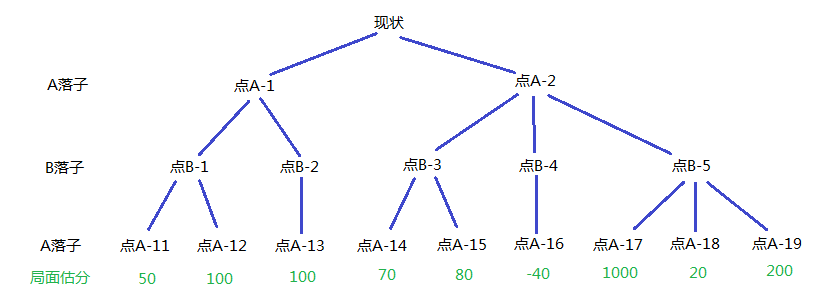这里考虑3步棋，那么如果A选择了第一步落在 A-1 点，B会落在哪一点呢？如果B落在 B-1 点，那么相应的A就会选择 A-12点，得分是100分，如果落在 B-2 点，A只能下在 A-13点，得分也是100分。也就是说，B下在B-1的预期得分是100分，下在B-2的预期得分也是100分，那么B随便在B-1和B-2间选一个就好了。这样一来，A-1点的预期得分就是100分。
类似的，可以知道，如果下在A-2点，那么B就会选择下在 B-4点，A-2的预期得分是 -40分。两者一对比，显然A-1 的预期得分更高，A应该选择落在 A-1点。
这个流程，我们可以在这张图上标示出来，点B-1标示B落完子，那么轮到A行动了，A是MAX选手，他会选择子节点中得分最高的进行上溯。而在A-1节点，是B在行动，他会选择子节点中得分最低的进行上溯，这个过程如下：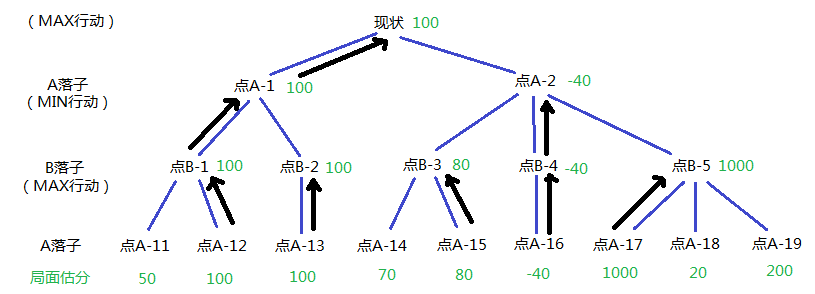如果AI按照这个方法选择最优点，那么就是所谓的MIN-MAX博弈算法。

4、alpha-beta剪枝
对上面的算法来说，其复杂度是呈指数增长的，一般来说，一个棋手可以下的落子位有几百个，那么即使只考虑2~3步棋，也是不小的开销，更不要说更高的深度了。实际上，我们不需要遍历树的每一条路径，就可以获得最优解，有些路径一看就没必要走，这就是剪枝的思想。
下面具体说alpha-beta剪枝
1）先说alpha，alpha是记录一个max节点（即图中的MAX行动节点）当前可选的最大值，如上图中的现状点，首先评估A-1点的得分，遍历其子树最终的达到得分是100，那么此时“现状节点”可选的最大值alpha就是100。
然后试探A-2节点。当获取到B-3节点的得分80之后，还有必要继续评估A-2的其他儿子吗？不需要了，因为B会选择最小得分点，那么A-2的最终得分，不会超过B-3节点的得分80分，所以剩下的B-4和B-5完全不用再看了，这样的剪枝，我们就称之为alpha-剪枝。
2）然后说beta，beta是记录一个MIN节点当前可选的最小值，以A-2点为例，当前是B行动，他要选取最小得分点。评估完B-3点后，当前的beta值是80，（顺便一提，此时ALPHA是100，应该发生alpha剪枝），然后继续评估B-4点，发现比原来的最小值还要小，那么更新beta到-40分。
然后试探B-5点，落子B-5后，先看看如果A下在A-17会怎样？结果局面得分是1000，超过了当前的beta值，这意味着，只要B下在B-5，那么A下过之后，局面的得分不会低于1000分（因为A会选择最优点），所以后面的A-18和A-19也完全不用再看了，我们称之为beta剪枝。

那么，将上面的两种情况同时使用起来，就是所谓的alpha-beta剪枝。在我实际的代码使用中，在MIN-MAX搜索深度为2的情况下，使用alpha-beta剪枝，大约能减少50%以上的计算量，还是非常有效的。

其实AI还有很大的优化空间，不过最近估计也没有什么时间去做优化了，以后掌握了新的算法再系统的改进吧。

展开全文• cocos creater 版本2.0.5 正常 运行的五子棋源码，仅作为学习使用
• 基于cocos2d-x 2.2.0的五子棋，仅仅作参考代码意义
• 、首先将spriteFrame为空的预制体chess加载到在15*15的棋盘上，并将其存进数组chesslist中 chesslist:{ default:[], type:[cc.node] }, chessBoard:function(){ //将chess预制体铺满棋this.ches...
一、首先将spriteFrame为空的预制体chess加载到在15*15的棋盘上，并将其存进数组chesslist中
        chesslist:{
default:[],
type:[cc.node]
},

    chessBoard:function(){
//将chess预制体铺满棋this.chessBoard()盘，然后点击时出发点击事件显示图片
for(var i=7;i>-8;i--)
{
for(var j=-7;j<8;j++)
{
//创建一个新的棋盘，将225个空棋子放入
var newCode=cc.instantiate(this.chessman)
newCode.setPosition(cc.v2(60*j,60*i))
//鼠标监听事件，每次下棋时进行一次判断
var self=this

newCode.on(cc.Node.EventType.TOUCH_END,function(event)
{
self.judgeOver()

});

this.chesslist.push(newCode)
}
}

},

二、在每个棋子上添加点击事件，并且实现黑字白字反复下子（将这个函数放在一个新的脚本中并且挂在在预制体中）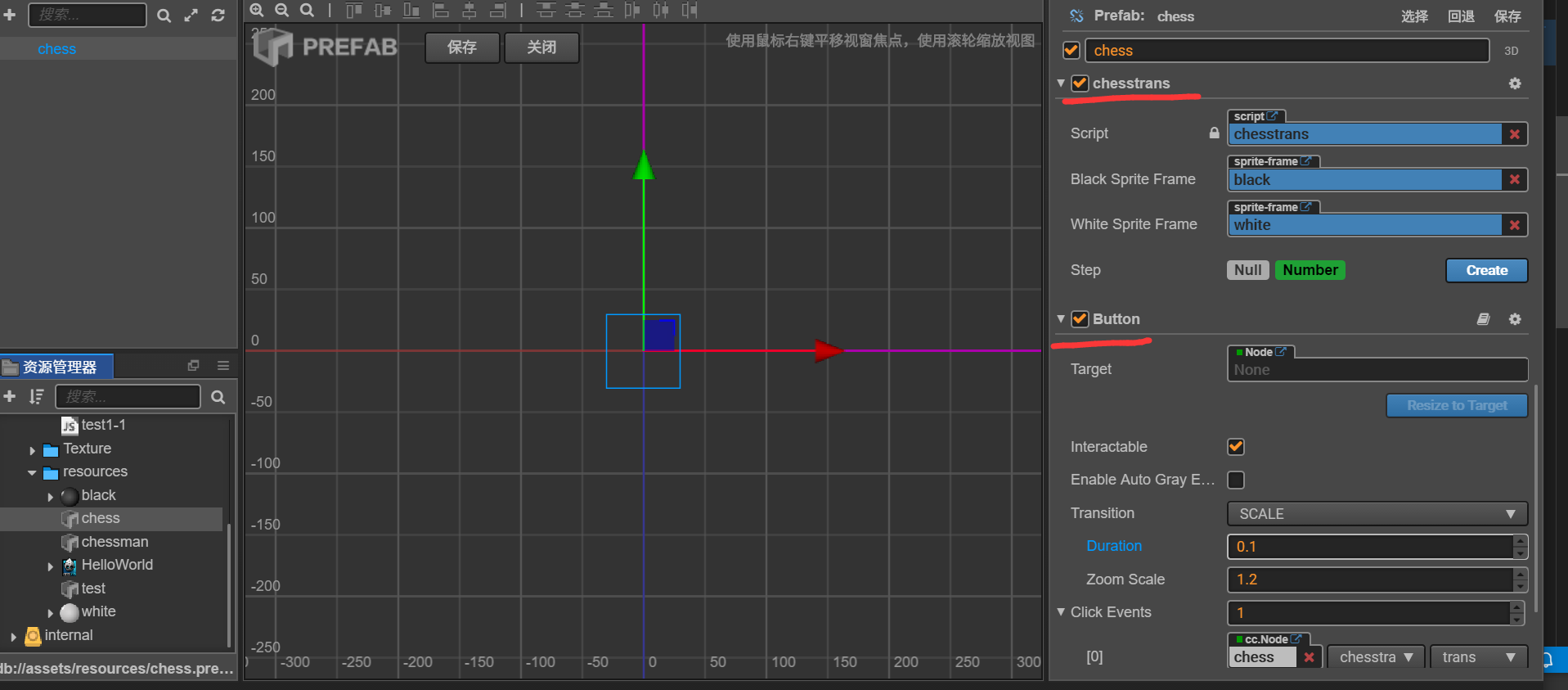trans:function(){

//落子的实现
var test=this
if(gamestate=='white' && this.getComponent(cc.Sprite).spriteFrame==null) //判断是否有棋子
{
this.getComponent(cc.Sprite).spriteFrame=this.whiteSpriteFrame  //将透明棋子变成白字
gamestate='black'   //每次点击时对棋子状态进行改变
cc.log(gamestate)
color=this.whiteSpriteFrame
chessbox.push(test)    //棋子下棋后入栈

}
else if(this.gamestate='black' && this.getComponent(cc.Sprite).spriteFrame==null)
{
this.getComponent(cc.Sprite).spriteFrame=this.blackSpriteFrame //将透明棋子变成黑字
gamestate='white'
cc.log(gamestate)
color=this.blackSpriteFrame
chessbox.push(test)   //棋子下棋后入栈

}
// this.getComponent(cc.Sprite).spriteFrame=this.blackSpriteFrame

//将棋子放进数组
},

三、判断胜负的函数
    judgeOver:function(){
var action=cc.moveTo(0.25,0,0)
var actionback=cc.moveTo(0.25,0,850)    //定义动作
//判断列
for(var i=0;i<11;i++)
{
for(var j=0;j<15;j++)
{
// cc.log(this.chesslist.getComponent(cc.Sprite).spriteFrame)
if(this.chesslist[(i+j*15)].getComponent(cc.Sprite).spriteFrame !== null && this.chesslist[(i+1+j*15)].getComponent(cc.Sprite).spriteFrame !== null
&& this.chesslist[(i+2+j*15)].getComponent(cc.Sprite).spriteFrame !== null && this.chesslist[(i+3+j*15)].getComponent(cc.Sprite).spriteFrame !== null
&& this.chesslist[(i+4+j*15)].getComponent(cc.Sprite).spriteFrame !== null)
{
var Over=cc.find("Canvas/background_1")

if(this.chesslist[(i*15+j)].getComponent(cc.Sprite).spriteFrame==this.whiteSpriteFrame)
{
//结束弹窗跳出
Over.runAction(action)
cc.find("Canvas/background_1/black_win").active=false

}
else
{
//结束弹窗跳出
Over.runAction(action)
cc.find("Canvas/background_1/white_win").active=false
}

}
// else cc.log("游戏继续")

}
}
//判断列

for(var i=0;i<15;i++)
{
for(var j=4;j<15;j++)
{
//判断是否有五个棋子连在一起

if(this.chesslist[(i+j*15)].getComponent(cc.Sprite).spriteFrame!==null && this.chesslist[(i+(j-1)*15)].getComponent(cc.Sprite).spriteFrame!==null
&& this.chesslist[(i+(j-2)*15)].getComponent(cc.Sprite).spriteFrame!==null && this.chesslist[(i+(j-3)*15)].getComponent(cc.Sprite).spriteFrame!==null
&& this.chesslist[(i+(j-4)*15)].getComponent(cc.Sprite).spriteFrame!==null)
{

var Over=cc.find("Canvas/background_1")

if(this.chesslist[(i+j*15)].getComponent(cc.Sprite).spriteFrame=this.whiteSpriteFrame)
{
//结束弹窗跳出
Over.runAction(action)
cc.find("Canvas/background_1/black_win").active=false

}
else
{
//结束弹窗跳出
Over.runAction(action)
cc.find("Canvas/background_1/white_win").active=false
}

}
// else cc.log("游戏继续")
}
}
//判断右斜方向
for(var i=0;i<11;i++)
{
for(var j=4;j<15;j++)
{
if(this.chesslist[(i+j*15)].getComponent(cc.Sprite).spriteFrame !== null && this.chesslist[(i+1+(j-1)*15)].getComponent(cc.Sprite).spriteFrame !== null
&& this.chesslist[(i+2+(j-2)*15)].getComponent(cc.Sprite).spriteFrame !== null && this.chesslist[(i+3+(j-3)*15)].getComponent(cc.Sprite).spriteFrame !== null
&& this.chesslist[(i+4+(j-4)*15)].getComponent(cc.Sprite).spriteFrame !== null)
{
var Over=cc.find("Canvas/background_1")
Over.runAction(action)
if(this.chesslist[(i+j*15)].getComponent(cc.Sprite).spriteFrame==this.whiteSpriteFrame)
{
//结束弹窗跳出
Over.runAction(action)
cc.find("Canvas/background_1/black_win").active=false
}
else
{
//结束弹窗跳出
Over.runAction(action)
cc.find("Canvas/background_1/white_win").active=false
}
}

}
}
//判断左斜方向
for(var i=4;i<15;i++)
{
for(var j=4;j<15;j++)
{
if(this.chesslist[(i+j*15)].getComponent(cc.Sprite).spriteFrame !== null && this.chesslist[(i-1+(j-1)*15)].getComponent(cc.Sprite).spriteFrame !== null
&& this.chesslist[(i-2+(j-2)*15)].getComponent(cc.Sprite).spriteFrame !== null && this.chesslist[(i-3+(j-3)*15)].getComponent(cc.Sprite).spriteFrame !== null
&& this.chesslist[(i-4+(j-4)*15)].getComponent(cc.Sprite).spriteFrame !== null)
{
var Over=cc.find("Canvas/background_1")
Over.runAction(action)
if(this.chesslist[(i+j*15)].getComponent(cc.Sprite).spriteFrame==this.whiteSpriteFrame)
{
//结束弹窗跳出
Over.runAction(action)
cc.find("Canvas/background_1/black_win").active=false
}
else
{
//结束弹窗跳出
Over.runAction(action)
cc.find("Canvas/background_1/white_win").active=false
}

}

}
}

},

四、悔棋的实现，我采用的是入栈arr.push()出栈arr.pop()的方法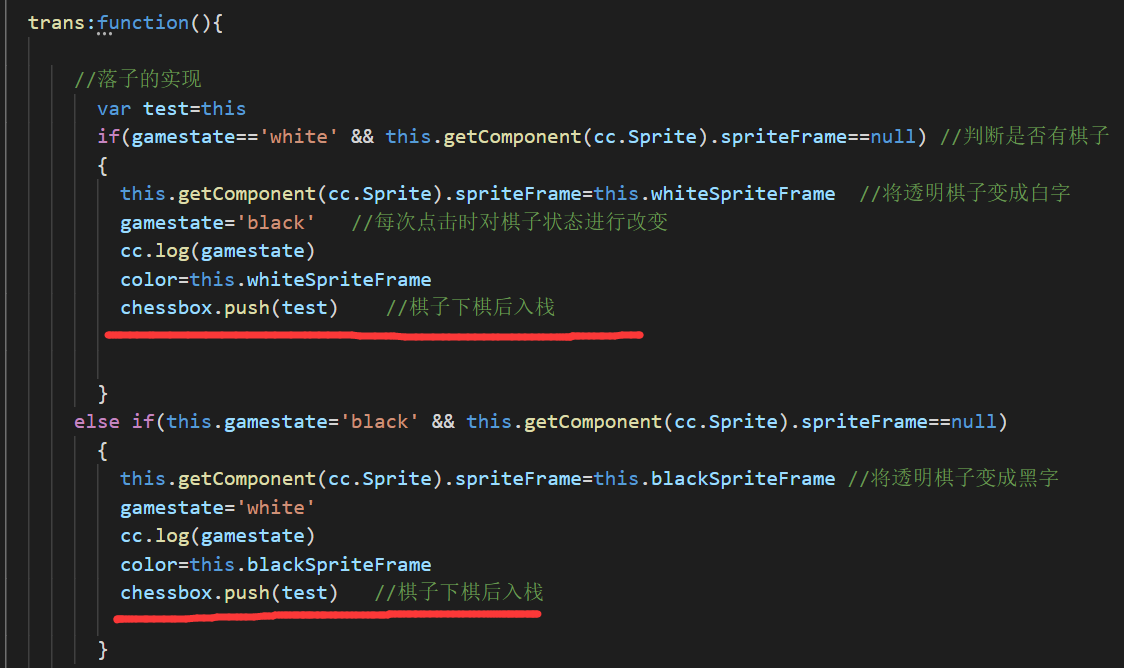在每次点击下棋子的时候将该棋子入栈，然后点击悔棋事件的时候通过arr.pop()获取最后一次下的棋子，然后获取该棋子的position属性，然后通过计算得到该棋子是第几个棋子
下面是悔棋事件的代码：
regret_chess:function(){

var chessman=chessbox.pop()
//获取到最后一次入栈的棋子，下面是获取其属性
cc.log(chessman)
//var chessmany=chessbox.pop().node.position.y
var chessmanx=chessman.node.position.y
var chessmany=chessman.node.position.x
var regret_chessman=(chessmany/60)+7+(7-chessmanx/60)*15
//出栈操作，获取最后一个入栈的节点，然后获取其position属性，并将其转换成为第几颗棋子

if(this.chesslist[regret_chessman].getComponent(cc.Sprite).spriteFrame==this.whiteSpriteFrame)
{
gamestate='white'
}
else
{
gamestate='black'
}
//判断最后一个是白棋还是黑棋，然后悔棋后仍然是该棋子下棋

this.chesslist[regret_chessman].getComponent(cc.Sprite).spriteFrame=null
//将该棋子变成空
},

现在这样人人对战，判断胜利以及悔棋已经实现。第二篇会将人机对战；第三篇会连接数据库实现有积分排行等功能。源码的话完成后会贴出来。


展开全文Cocos-creator 人机对战
• 五子棋人机算法 分析：首先在玩家下完子之后，需要ai下棋。那么ai应该是去拦截玩家的棋子或者使自己棋子更容易形成五连，所以在ai眼里并不是所有棋盘上的空位置都是一样的。很定有优先考虑的位置。那么我们将采用对...
五子棋人机算法
分析：首先在玩家下完子之后，需要ai下棋。那么ai应该是去拦截玩家的棋子或者使自己棋子更容易形成五连，所以在ai眼里并不是所有棋盘上的空位置都是一样的。很定有优先考虑的位置。那么我们将采用对棋盘空位置进行赋分的方法来进行区别每个位置。
思路：每次下子后，遍历整个棋盘，对棋盘的每个空位置进行赋分------然后找到分值最好的位------ai进行落子
方法一：对每个空位置的八个方向进行
下面我们用这个赋分的规则：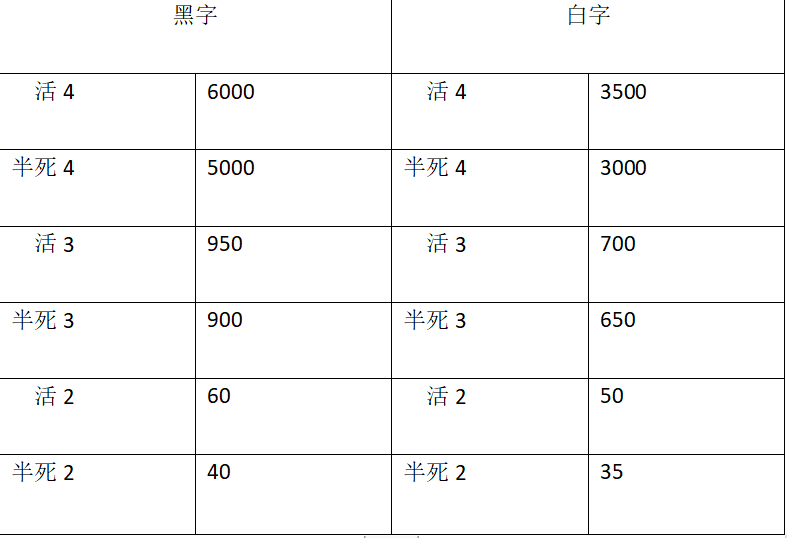这里我们采用对每个空位的上、下、左、右、左上、左下、右上、右下八个方向判断棋子的分布情况。
下面的用c++实现的源码，因为creator是脚本文件所以硬生生从项目中拉出来可能有些地方会有点生硬：
#define  _CRT_SECURE_NO_WARNINGS
#include<stdio.h>
#include<iostream>
using namespace std;
#include <stdlib.h>
#include<stdlib.h>
#define black 1
#define white 0
char qip;
char black_flag = '*';
char white_flag = '#';
int qinxingpc = { 0 };
int qinxingplayer = { 0 };
int player = black;
void jifen();
int jieguo(int left, int right, int count, int k, char num);
int shu(int hang, int lie, char whoflag);
int heng(int hang, int lie, char whoflag);
int zuoxie(int hang, int lie, char whoflag);
int youxie(int hang, int lie, char whoflag);
void init_qip();
void draw_qip();
int iswin();

int main()
{
int result;
int nums;
init_qip();
draw_qip();   //构建棋盘
for (nums = 0; nums < 225; nums++)
{
if (player == black)
{
player = white;
if (!result) break;
}            //玩家下棋
else
{
player = black;
}            //ai下棋
draw_qip();
if (iswin( ))
{
if (player == white)

{
printf("玩家1(%c) is winner.\n", white_flag);
}
else
{
printf("ai(%c) is winner.\n", black_flag);
}
break;
}
}
}
void init_qip()
{
int i = 0;
int j = 0;
for (i = 0; i <15; i++)
{
for (j = 0; j < 15; j++)
{
qip[i][j] = '.';
}
}      //构建棋盘15*15
}
void draw_qip()
{
int i = 0, j = 0, k = 0;
system("cls");                          //清零//
printf("    X--------------------------->\n");  //x轴
printf("    ");
for (k = 0; k < 15; k++)
{
if (k >= 0 && k <= 8)
{
printf("%d ", k + 1);
}
else
{
printf("%d", k + 1);
}
}
putchar('\n');
for (i = 0; i < 15; i++)
{
printf("Y");
if (i >= 0 && i <= 8)
{
printf("0%d ", i + 1);
}
else
{
printf("%d ", i + 1);
}
for (j = 0; j <15; j++)
{
putchar(qip[i][j]);
if (j<14)  putchar(' ');
}
putchar('\n');
}
}
int heng(int hang, int lie, char whoflag)
{
int spacenum = 0;//空白数
int count = 1;//几连
int x = hang;
int y = lie;
int liveleft = 0;  //0 1表示活死
int liveright = 0;
if (qip[hang][lie] != '.')
{
return 0;
}          //该位置不判断
while (y > 0 && (qip[x][y - 1] == '.' || qip[x][y - 1] == whoflag))
{
if (qip[x][y - 1] == '.'&&spacenum < 1)
{
if (qip[x][y - 2] != whoflag)
{
liveleft = 1;
break;     //如果该子左边第二个不是该棋子，则跳出
}
spacenum++;
y--;   //如果左边第一个为空的话，则空白数加1 ，左活
}
else if (qip[x][y - 1] == whoflag)
{
y--;
count++;
}                    //如果左边第一个有棋子，则连子数加1
else
{
liveleft = 1;
break;
}        //其他情况，左活
}                      //while判断该位置左边的连子情况
{
spacenum = 0;  //如果左边无连子，则不做判断
}
//y = lie;
while (y < 14 && (qip[x][y + 1] == '.' || qip[x][y + 1] == whoflag))
{
if (qip[x][y + 1] == '.'&&spacenum < 1)
{
if (qip[x][y + 2] != whoflag)
{
liveright = 1;
break;
}
spacenum++;
y++;
}                    //如果右边相邻两个都为空的，则空白数加1，右活
else if (qip[x][y + 1] == '.'&&spacenum>0)
{
liveright = 1;
break;
}
else
{
y++;
count++;
}
}
return jieguo(liveleft, liveright, count, spacenum, whoflag);

}
int shu(int hang, int lie, char whoflag)
{
int spacenum = 0;
int count = 1;
int x = hang;
int y = lie;
int liveleft = 0;
int liveright = 0;
if (qip[hang][lie] != '.')
{
return 0;
}
while (x > 0 && (qip[x - 1][y] == '.' || qip[x - 1][y] == whoflag))
{
if (qip[x - 1][y] == '.'&&spacenum < 1)
{
if (qip[x - 2][y] != whoflag)
{
liveleft = 1;
break;
}
spacenum++;
x--;
}
else if (qip[x - 1][y] == whoflag)
{
x--;
count++;
}
else
{
liveleft = 1;
break;
}
}
{
spacenum = 0;
}
x = hang;
while (x<14 && (qip[x + 1][y] == '.' || qip[x + 1][y] == whoflag))
{
if (qip[x + 1][y] == '.' && spacenum<1)
{
if (qip[x + 2][y] != whoflag)
{
liveright = 1;
break;
}
spacenum++;
x++;
}
else if (qip[x + 1][y] == '.' && spacenum>0)
{
liveright = 1;
break;
}
else
{
x++;
count++;
}
}
return jieguo(liveleft, liveright, count, spacenum, whoflag);
}
int zuoxie(int hang, int lie, char whoflag)
{
int spacenum = 0;
int count = 1;
int x = hang;
int y = lie;
int liveleft = 0;
int liveright = 0;
if (qip[hang][lie] != '.')
{
return 0;
}
while (x<14 && y>0 && (qip[x + 1][y - 1] == '.' || qip[x + 1][y - 1] == whoflag))
{
if (qip[x + 1][y - 1] == '.' && spacenum<1)
{
if (qip[x + 2][y - 2] != whoflag)
{
liveleft = 1;
break;
}
spacenum++;
x++;
y--;
}
else if (qip[x + 1][y - 1] == whoflag)
{
x++;
y--;
count++;
}
else
{
liveleft = 1;
break;
}
}
if
{
spacenum = 0;
}
x = hang;    y = lie;
while (x>0 && y<14 && (qip[x - 1][y + 1] == '.' || qip[x - 1][y + 1] == whoflag))
{
if (qip[x - 1][y + 1] == '.' && spacenum<1)
{
if (qip[x - 2][y + 2] != whoflag)
{
liveright = 1;
break;
}
spacenum++;
x--;
y++;
}
else if (qip[x - 1][y + 1] == '.' && spacenum>0)
{
liveright = 1;
break;
}
else
{
x--;
y++;
count++;
}
}
return jieguo(liveleft, liveright, count, spacenum, whoflag);
}
int youxie(int hang, int lie, char whoflag)
{
int spacenum = 0;
int count = 1;
int x = hang;
int y = lie;
int liveleft = 0;
int liveright = 0;
if (qip[hang][lie] != '.')
{
return 0;
}
while (x>0 && y>0 && (qip[x - 1][y - 1] == '.' || qip[x - 1][y - 1] == whoflag))
{
if (qip[x - 1][y - 1] == '.' && spacenum<1)
{
if (qip[x - 2][y - 2] != whoflag)
{
liveleft = 1;
break;
}
spacenum++;
x--;
y--;
}
else if (qip[x - 1][y - 1] == whoflag)
{
x--;
y--;
count++;
}
else
{
liveleft = 1;
break;
}
}
{
spacenum = 0;
}
x = hang;    y = lie;
while (x<14 && y<14 && (qip[x + 1][y + 1] == '.' || qip[x + 1][y + 1] == whoflag))
{
if (qip[x + 1][y + 1] == '.' && spacenum<1)
{
if (qip[x + 2][y + 2] != whoflag)
{
liveright = 1;
break;
}
spacenum++;
x++;
y++;
}
else if (qip[x + 1][y + 1] == '.' && spacenum>0)
{
liveright = 1;
break;
}
else
{
x++;
y++;
count++;
}
}
return jieguo(liveleft, liveright, count, spacenum, whoflag);
}
int jieguo(int left, int right, int count, int k, char num)
{
if (count == 1)                                  //对1连打分
{
return 1;
}
else if (count == 2)                              //对2连打分
{
if (left&&right)                               //活2
{
if (k == 0)
{
return num == black_flag ? 60 : 50;   //有两空位及以上
}
else
{
return num == black_flag ? 40 : 35;   //仅有一空位
}
}
else if (!left&&!right)                     //死2
{
return  1;
}

else                                        //半死2
{
return 10;
}
}
else if (count == 3)
{
if (left&&right)                       //活3
{
if (k == 0)
{
return num == black_flag ? 950 : 700;
}
else
{
return num == black_flag ? 900 : 650;
}
}
else if (!left&&!right)                   //死3
{
return 1;
}
else                                     //半死3
{
return 100;
}
}
else if (count == 4)
{
if (left&&right)                  //活4
{
if (k == 0)
{
return num == black_flag ? 6000 : 3500;
}
else
{
return num == black_flag ? 5000 : 3000;
}
}
else if (!left&&!right)           //死4
{
return 1;
}
else                            //半死4
{
if (k == 0)
{
return num == black_flag ? 4000 : 800;
}
else
{
return num == black_flag ? 3600 : 960;
}
}
}
else {
if (k == 0)
{
return num == black_flag ? 20000 : 15000;
}
else
{
return num == black_flag ? 10000 : 3300;
}
}
}
{
jifen();
int count = 0;
int hang = 0;
int lie = 0;
int i = 0;
int j = 0;
for (i = 0; i< 15; i++)
{
for (j = 0; j< 15; j++)
{
if (qinxingpc[i][j] > count)
{
count = qinxingpc[i][j];
hang = i;
lie = j;
}
if (qinxingplayer[i][j] > count)
{
count = qinxingplayer[i][j];
hang = i;
lie = j;
}
}                                 //将判断出分值最高的坐标输出出来
}
printf("qinxingPC[%d][%d]:%d\n", hang, lie, qinxingpc[hang][lie]);
printf("qinxingPlayer[%d][%d]:%d\n", hang, lie, qinxingplayer[hang][lie]);
if (qip[hang][lie] == '.')
{
qip[hang][lie] = black_flag;
}                                          //对分值最高的位置下棋
}
{
int x, y;
int res;
cout << "input" << "x" << " " << "y";
cin >> y >> x;
/*printf("input y x:");
scanf("%d %d", &x, &y);*/
if (x<0 || y<0 || x>15 || y>15)
{
printf("input error,again\n");               //判断棋子是否落在棋盘上
while ((getchar()) != '\n');
if (res == 1) return 1;
}
x--;
y--;
if (qip[x][y] == '.')
{
if (player == white)
{
qip[x][y] = white_flag;
}
}
else
{
printf("input error2,again\n");               //判断是否重子
while ((getchar()) != '\n');
if (res == 1) return 1;
}
return 1;
}
void jifen()
{
int n = 0;
int m = 0;
for (n = 0; n<15; n++)
{
for (m = 0; m<15; m++)
{
qinxingpc[n][m] = heng(n, m, black_flag) + shu(n, m, black_flag) + zuoxie(n, m, black_flag) + youxie(n, m, black_flag);            //计算ai在该位置的总分
qinxingplayer[n][m] = heng(n, m, white_flag) + shu(n, m, white_flag) + zuoxie(n, m, white_flag) + youxie(n, m, white_flag);         //计算player在该位置的总分
}
}
}
int iswin()
{
char m;

int i, j;
if (player == white)   m = white_flag;
else m = black_flag;
for (i = 0; i <15; i++)
{
for (j = 0; j < 15; j++)
{
if (qip[i][j] == m)
{
if ((i + 4) <15)
{
if (qip[i + 1][j] == m && qip[i + 2][j] == m && qip[i + 3][j] == m && qip[i + 4][j] == m) return 1;
}
if ((j + 4) < 15)
{
if (qip[i][j + 1] == m && qip[i][j + 2] == m && qip[i][j + 3] == m &&qip[i][j + 4] == m) return 1;
}
if
((i + 4) <15 && (j + 4) < 15)
{
if (qip[i + 1][j + 1] == m && qip[i + 2][j + 2] == m && qip[i + 3][j + 3] == m && qip[i + 4][j + 4] == m) return 1;
}
if ((i + 4) < 15 && (j - 4) >= 0)
{
if (qip[i + 1][j - 1] == m && qip[i + 2][j - 2] == m && qip[i + 3][j - 3] == m && qip[i + 4][j - 4] == m) return 1;
}                                                                                                                                   //一个子向4个方向来判断是否有5连
}
}
}
return 0;
}



展开全文c++五子棋算法 creator
• 这是多月前的，只是现在想起了，AI低，博弈树那块没（我也没兴趣继续了）。 环境：win7 cocos2d-x 2.2.0 vs2010 是python建立工程的（参考我的上篇...
这是个多月前写的，只是现在想起了，AI低，博弈树那块没写（我也没兴趣继续写了）。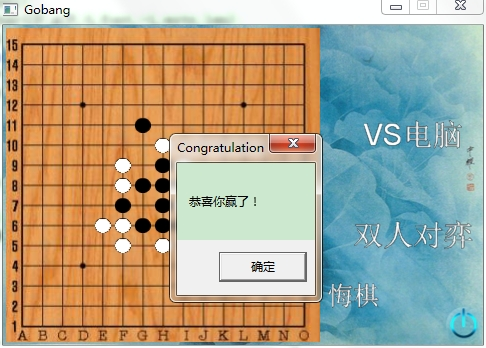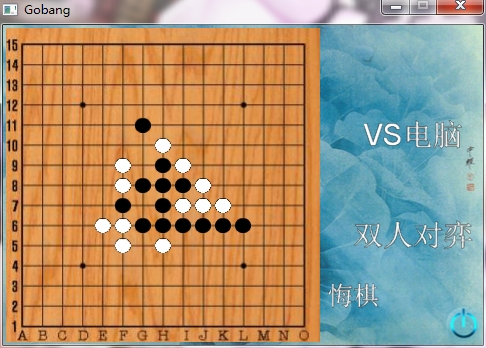环境：win7       cocos2d-x 2.2.0      vs2010
是用python建立工程的（参考我的上一篇http://blog.csdn.net/niteip/article/details/17558727）
我试了下，项目只能放在cocos的projects文件夹下，变动将导致编译失败（我不知道为什么，但cocos项目组说这是为了跨平台的考虑，我在想这真的是方便跨平台吗）

我写的过程，是先界面、逻辑、数据结构，最后AI。难度不大，最复杂的一块AI只是遍历考虑落点权值，博弈树 α-β剪枝就算了。无游戏性，仅仅作为参考代码意义。


展开全文cocos2d-x
• 主要是有8个方向，代码有点长，其实思路还是挺简单的int PlayScene::IsWin(qiziSprite *q,int direction,int c) //判断胜负 三个参数，第一个是当前棋子，第二个寻找方向 第三个数量 { bool upPe

算法思路：下棋之后，把自己想象成那颗新棋子，假定为a，现在寻找a周围八格的小伙伴，找到了b，那么现在我们走到了b，然后b不需要找周围八格的小伙伴了，只需要找a→b方向的小伙伴即可，如果还能找到c，就继续在c找a→b方向的小伙伴，依次找下去，直至找不到小伙伴了，这时候就返回小伙伴的个数。
当然，这样的情况不是完全的，因为在小伙伴a→b的反方向，即b→a，可能会有小伙伴在那里，所以我们需要在找完a的一边之后锁定其反方向寻找小伙伴，最后返回两个方向的总和。

主要是有8个方向，代码有点长，其实思路还是挺简单的

void PlayScene::IsWin(const qiziSprite *q,int direction,int &c) //判断胜负 三个参数，第一个是当前棋子，第二个寻找方向 第三个数量
{

CCLOG("now %d",c);
Point NowPoint = q->getlocate();
int* count =  &c;
//if(q周围8格有同一棋子)
//寻找该方向的另一棋子(反方向亦可)
//直到寻找到5个相同方向的棋子为止
for(qiziSprite *s : _Sprite)
{
if(*count == 5) break;
if(s->getType()==q->getType())
{
Point NextPoint = s->getlocate();
if((direction==-1||direction==RIGHT)
&&NowPoint == Point(NextPoint.x-1,NextPoint.y))  //右边
{
IsWin(s,RIGHT,++*count);
if(direction == -1) direction=LEFT; //设置反方向
else break; //如果已经规定方向，就没必要遍历下去其他的了，直接中断，节约时间

}
if((direction==-1||direction==UPPERRIGHT)
&&NowPoint == Point(NextPoint.x-1,NextPoint.y-1)) //右上
{
IsWin(s,UPPERRIGHT,++*count);
if(direction == -1) direction=BOTTOMLEFT;
else break;
}
if((direction==-1||direction==UPPER)
&&NowPoint == Point(NextPoint.x,NextPoint.y-1)) //上方
{
IsWin(s,UPPER,++*count);
if(direction == -1) direction=BOTTOM;
else break;
}
if((direction==-1||direction==UPPERLEFT)
&&NowPoint == Point(NextPoint.x+1,NextPoint.y-1)) //左上
{
IsWin(s,UPPERLEFT,++*count);
if(direction == -1) direction=BOTTOMRIGHT;
else break;
}
if((direction==-1||direction==LEFT)
&&NowPoint == Point(NextPoint.x+1,NextPoint.y)) //左方
{
IsWin(s,LEFT,++*count);
if(direction == -1) direction=RIGHT;
else break;
}
if((direction==-1||direction==BOTTOMLEFT)
&&NowPoint == Point(NextPoint.x+1,NextPoint.y+1)) //左下
{
IsWin(s,BOTTOMLEFT,++*count);
if(direction == -1) direction=UPPERRIGHT;
else break;
}
if((direction==-1||direction==BOTTOM)
&&NowPoint == Point(NextPoint.x,NextPoint.y+1)) //下方
{
IsWin(s,BOTTOM,++*count);
if(direction == -1) direction=UPPER;
else break;
}
if((direction==-1||direction==BOTTOMRIGHT)
&&NowPoint == Point(NextPoint.x-1,NextPoint.y+1)) //右下
{
IsWin(s,BOTTOMRIGHT,++*count);
if(direction == -1) direction=UPPERLEFT;
else break;
}
}
}
}
但是以上方法还是有问题的，首先，下图的情况只能判断出3个白字，为什么呢？因为第一次寻找的时候首先找到的是左边的白子，然后锁定右边寻找下去，左下的白子被忽略掉了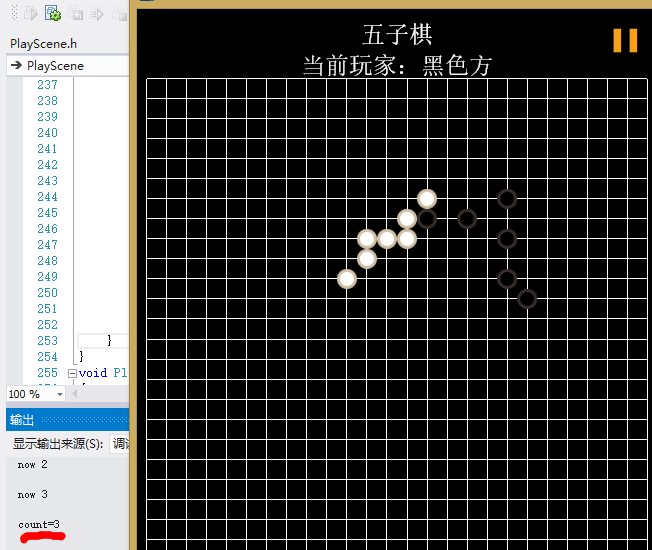所以解决方法呢，在下面，首先规定好4个方向，查找4个方向的棋子，选出棋子数最多的

//判断胜利
void PlayScene::IsWin(const qiziSprite *q,int direction,int &c) //判断胜负 三个参数，第一个是当前棋子，第二个寻找方向 第三个数量
{

CCLOG("direction %d now %d",direction,c);
Point NowPoint = q->getlocate();
int* count =  &c;
int num = {1}; //四个方向
//if(q周围8格有同一棋子)
//寻找该方向的另一棋子(反方向亦可)
//直到寻找到5个相同方向的棋子为止
for(qiziSprite *s : _Sprite)
{
if(*count == 5) break;
if(s->getType()==q->getType())
{
Point NextPoint = s->getlocate();
if((direction==-1||direction==RIGHT)
&&NowPoint == Point(NextPoint.x-1,NextPoint.y))  //右边
{
IsWin(s,RIGHT,++num);
if(direction == -1)
IsWin(s,LEFT,++num);//设置反方向寻找
else break;

}
if((direction==-1||direction==UPPERRIGHT)
&&NowPoint == Point(NextPoint.x-1,NextPoint.y-1)) //右上
{
IsWin(s,UPPERRIGHT,++num);
if(direction == -1)
IsWin(s,BOTTOMLEFT,++num);
else break;
}
if((direction==-1||direction==UPPER)
&&NowPoint == Point(NextPoint.x,NextPoint.y-1)) //上方
{
IsWin(s,UPPER,++num);
if(direction == -1)
IsWin(s,BOTTOM,++num);
else break;
}
if((direction==-1||direction==UPPERLEFT)
&&NowPoint == Point(NextPoint.x+1,NextPoint.y-1)) //左上
{
IsWin(s,UPPERLEFT,++num);
if(direction == -1)
IsWin(s,BOTTOMRIGHT,++num);
else break;
}
if((direction==-1||direction==LEFT)
&&NowPoint == Point(NextPoint.x+1,NextPoint.y)) //左方
{
IsWin(s,LEFT,++num);
if(direction == -1)
IsWin(s,RIGHT,++num);
else break;
}
if((direction==-1||direction==BOTTOMLEFT)
&&NowPoint == Point(NextPoint.x+1,NextPoint.y+1)) //左下
{
IsWin(s,BOTTOMLEFT,++num);
if(direction == -1)
IsWin(s,UPPERRIGHT,++num);
else break;
}
if((direction==-1||direction==BOTTOM)
&&NowPoint == Point(NextPoint.x,NextPoint.y+1)) //下方
{
IsWin(s,BOTTOM,++num);
if(direction == -1)
IsWin(s,UPPER,++num);
else break;
}
if((direction==-1||direction==BOTTOMRIGHT)
&&NowPoint == Point(NextPoint.x-1,NextPoint.y+1)) //右下
{
IsWin(s,BOTTOMRIGHT,++num);
if(direction == -1)
IsWin(s,UPPERLEFT,++num);
else break;
}
}
if(direction == -1)  //在最高层选出最多的
for(int n : num)
if( *count < n) *count = n; //选出最多的
}
}

展开全文• 这里自定义目录标题欢迎使用Markdown编辑器新的改变功能快捷键合理的创建标题，有助于目录的生成如何改变文本的样式插入链接与图片如何插入一段漂亮的代码片生成一个适合你的列表创建一个表格设定内容居中、居左、...
• 在15*15的棋盘上每一个可下棋子的地方都放置一个“隐形的棋子”,当要在某个位置下子时就将该位置的棋子显示出来，在判断输赢逻辑里，我们根据这225个”隐形棋子”的状态(黑，白，无)判断输赢 涉及知识点 场景切换 ...算法 闭包
• 五子棋最大的难点就是自己落子后，电脑在什么位置落子， 我这里了 http://blog.csdn.net/onezeros/article/details/5542379 这篇文章里的第种算法 己方落子的话，就触摸棋盘，然后判断触摸点离棋盘上的哪个点...cocos2d-x 算法
• cocos creator游戏合集，里面有五子棋、2048、拼图、俄罗斯方块、连连看、打砖块等。方便学习cocos跨平台游戏的开发，学习提高游戏编程的逻辑能力。
• 五子棋问题归结为一个寻找某状态下的棋盘的最佳落子点的问题，所以我们不如使用一个整型二维数组来代表棋盘状态，每个元素这一点的状态，0表示该处无子，1为黑子，2为白子。将下棋时棋落在哪一点 的决策标准抽象...cocos2d-x
• 游戏规则，有一个10*10的棋盘，里面有六种颜色的珠子，点击棋盘的珠子可以移动到任意有开放路径的位置，如果横、竖、斜、反斜可以连接相同颜色珠子数大于等于5个，就可以消除。如果移动珠子后没有消除珠子，则会增3...cocos2d-x
• 今天我想要分享一下我... 那我的五子棋是15*15的大小（一般也就是这样的一个大小）。我的AI算法要求每一次落子之后都要去计算每一个空暇的位置的“分值”，简单的说，我们需要一个存放棋子的数组，表示是否存放了棋子cocos2d-x 五子棋AI
• 为什突然做这个，因为这是个笔试题，拖了一个月才（最近终于闲了O(∩_∩)O），废话不多说，说说这个题吧 题目要求 编写一个单机【五子棋】游戏，要求如下： 1.使用原生技术实现，兼容 Chrome 浏览器即可。 2.实现...
• public class MainClass { // 创建棋盘 public void Create(char board[][]) { for (int i = 0; i &lt; 10; i++) { for (int j = 0; j &lt; 10; j++) { board[i][j] = '+';... i ...
• 上周跟大家分享了AS3.0 实现五子棋悔棋和复盘功能的实现，这周我做了五子棋AI功能。接下来跟大家分享下我关于五子棋AI的思路:  第步: 遍历棋盘中所有棋子坐标，搜索出该坐标四方向周围的棋局并记录在数组中...cocos2d-x
...